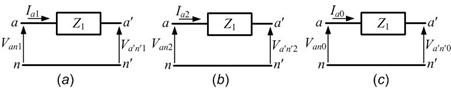## Zero Sequence Impedance of Transmission Line:

Zero Sequence Impedance of Transmission Line – A fully transposed three-phase line is completely symmetrical and therefore the per phase impedance offered by it is independent of the phase sequence of a balanced set of currents. In other words, the Sequence Impedance of Transmission Line offered by it to positive and negative sequence currents are identical. The expression for its per phase inductive reactance accounting for both self and mutual linkages has been derived.

When only zero sequence currents flow in a transmission line, the currents in each phase are identical in both magnitude and phase angle. Part of these currents return via the ground, while the rest return through the overhead ground wires. The ground wires being grounded at several towers, the return currents in the ground wires are not necessarily uniform along the entire length.The flow of zero sequence currents through the transmission lines, ground wires and ground creates a magnetic field pattern which is very different from that caused by the flow of positive or negative sequence currents where the currents have a phase difference of 120° and the return current is zero.

The zero sequence impedance of transmission line also accounts for the ground impedance (Z0 = Zl0 + 3Zg0). Since the ground impedance heavily depends on soil conditions, it is essential to make some simplifying assumptions to obtain analytical results.

The zero sequence impedance of transmission lines usually ranges from 2 to 3.5 times the positive sequence impedance. This ratio is on the higher side for double circuit lines without ground wires.

Scroll to Top